International
Tables for
Crystallography
Volume C
Mathematical, physical and chemical tables
Edited by E. Prince

International Tables for Crystallography (2006). Vol. C, ch. 9.8, p. 913

## Section 9.8.1.5. Occupation modulation

T. Janssen,a A. Janner,a A. Looijenga-Vosb and P. M. de Wolffc

aInstitute for Theoretical Physics, University of Nijmegen, Toernooiveld, NL-6525 ED Nijmegen, The Netherlands,bRoland Holstlaan 908, NL-2624 JK Delft, The Netherlands, and cMeermanstraat 126, 2614 AM, Delft, The Netherlands

#### 9.8.1.5. Occupation modulation

| top | pdf |

Another type of modulation, the occupation modulation, can be treated in a way similar to the displacive modulation. As an example consider an alloy where the positions of the basic structure have space-group symmetry, but are statistically occupied by either of two types of atoms. Suppose that the position r is occupied by an atom of type A with probability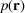and by one of type B with probability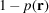and that p is periodic. The probability of finding an A atom at site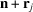is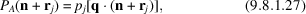with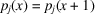. In this case, the structure factor becomes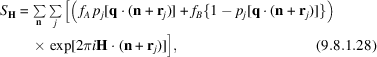where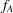and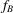are the atomic scattering factors. Because of the periodicity, one has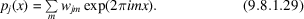Hence,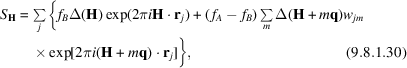where Δ(H) is the sum of δ functions over the reciprocal lattice of the basic structure: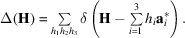Consequently, the diffraction peaks occur at positions H given by (9.8.1.7). For a simple sinusoidal modulation [m = ±1 in (9.8.1.29)], there are only main reflections and first-order satellites (m = ±1). One may introduce an additional coordinate t and generalize (9.8.1.27)to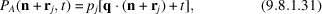which has (3 + 1)-dimensional space-group symmetry. Generalization to more complex modulation cases is then straightforward.